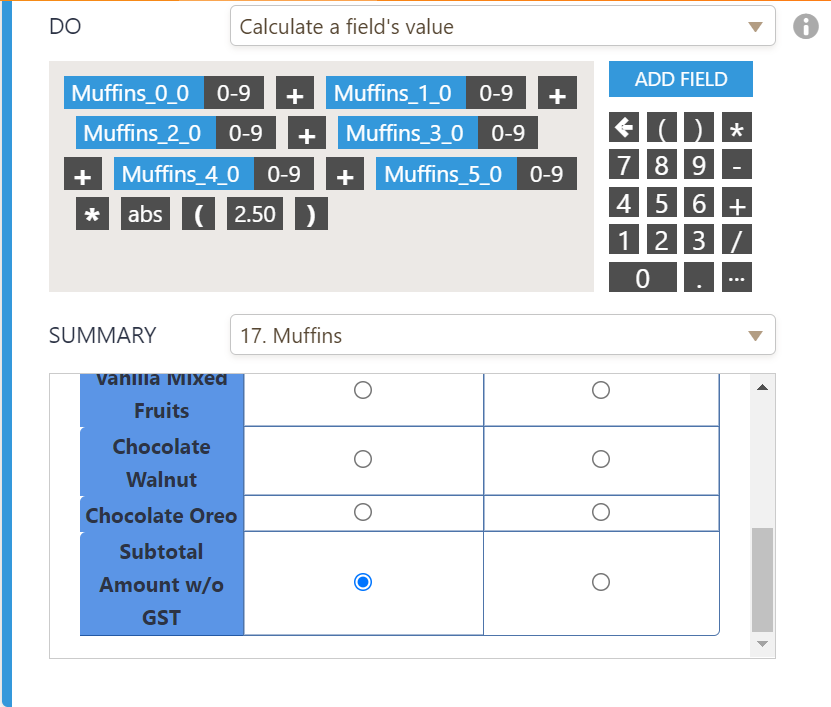# How do I multiple a decimal number with input table?

•marketing02
Asked on June 28, 2021 at 03:37 AM

Hi, I've tried to calculate, but cant get the right calculation.

The amount reflected in Subtotal Amt w/o GST is the total of summed fields.

Page URL:
https://form.jotform.com/211752249882462•gerardw
Answered on June 28, 2021 at 06:43 AM

With respect to the current conditional calculation, the total is shown correctly on the input table:Is item each valued at \$2.50?

Then you will need to add () in the calculation as shown:Please check and let us know.

•marketing02
Answered on June 28, 2021 at 10:16 PM

Yes, it does work. Tq so much.

But may I bother u again, if I use the Form Calculation to calculate GST using below calculation will it be possible? as of now, I've tried but no calculation done.

Or do I need to is the condition to do the calculation?•gerardw
Answered on June 28, 2021 at 11:31 PM

You can also use the widget instead of conditions to calculate.

Replace 7% with 0.07 and check:Do check and let us know.

•marketing02
Answered on June 29, 2021 at 12:43 AM

Yes. It really work. Tq so much.

Btw, I send a new support request. Please assist.

•gerardw
Answered on June 29, 2021 at 03:01 AM